# Kirchhoff’s Voltage Law Examples

The Kirchhoff’s Laws are very useful in solving electrical networks which may not be easily solved by Ohm’s Law. In this article, I will describe these laws and will show some of Kirchhoff’s voltage law examples to make these laws easily understandable. Kirchhoff’s Laws, two in number, are as follows:

• Kirchhoff’s Current Law
• Kirchhoff’s Voltage Law or Kirchhoff’s Loop Law

## Kirchhoff’s Current Law

This law states that “The algebraic sum of all the currents meeting at a point or a junction in an electric circuit is zero”.

• Consider five wires carrying current I1, I2, I3, I4, I5 meeting at a point O.
• To take the algebraic sum, the sigh of the current is to be considered.
• If we take the flow of current towards point O as positive, then the flow of current away from point O will be negative.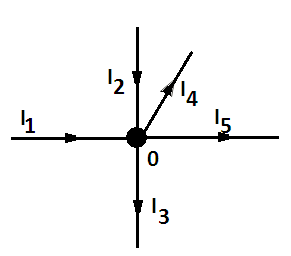Applying Kirchhoff’s Current Law,

The algebraic sum of current at point O = zero

i.e. I1 + I2 + (-I3) + (-I4) + (-I5) = 0

or I1 + I2 = I3 + I4 + I5

i.e. Incoming currents = Outgoing currents

Hence, Kirchhoff’s Current Law can also be stated as, “The sum of incoming currents is equal to the sum of outgoing currents at a point or junction in an electric circuit”.

## Kirchhoff’s Voltage Law

This law relates to voltages and applied to a closed circuit or mesh, therefore, it is also known as Kirchhoff’s Loop Law.

This law states that “In any closed circuit or mesh, the algebraic sum of all the EMF’s plus the algebraic sum of voltage drops is zero”.

## Sign Conventions

Whenever Kirchhoff’s Voltage Law is to be applied to any closed circuit or a mesh algebraic sum of EMF’s and voltage drops are to be considered. Therefore, EMF’s and voltage drops must be given a proper sign.

A rise in potential should be considered positive while fall in potential should be considered negative.

## Signs to be given to EMF’s

While tracing any circuit, if we go from negative terminal of a battery or source of EMF to the positive terminal, there is a rise in potential and it should be given a +ve sign.

Whereas, if we go from positive terminal to negative terminal, there is a fall in potential and it should be given –ve sign.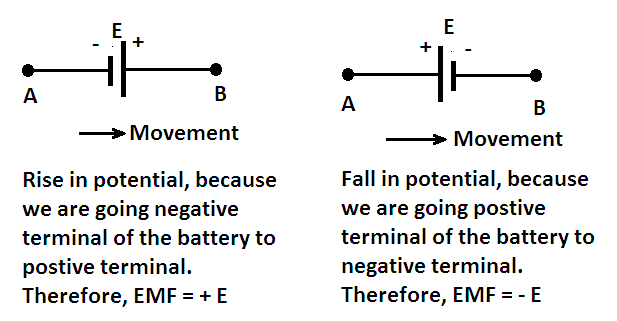It is worthwhile to note that sign of EMF’s is independent of the direction of flow of current.

## Signs to be given to Voltage Drops

When current flows through a resistance, there is a voltage drop (IR).

If we go in the direction of flow of current, a negative sign should be given to the voltage drop (fall in potential) as current flows from higher potential to lower potential.

However, if we go in the opposite direction to the flow of current a positive sign should be given to the voltage drop (rise in potential).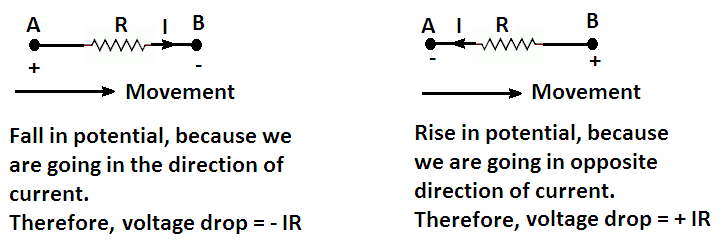It is worthwhile to note that the sign of voltage drop depends upon the direction of flow of current through the resistance.

## Steps to solve circuits by Kirchhoff’s Voltage Law

• Mark the direction of flow of current in various branches of the circuit according to Kirchhoff’s Current Law.
• Choose as many numbers of closed circuits as the number of unknown quantities.
• Find the algebraic sum of voltage drops and EMF’s in that circuit and put their sum equal to zero.
• After solving the problem, if the calculated value of the current has a +ve sign, it indicates that the direction assumed is correct. If it has –ve sign, it means that the actual direction of flow of current is opposite to that of assumed direction.

# Kirchhoff’s Voltage Law Examples

Example: Apply Kirchhoff’s voltage law to the adjoining circuit and calculate the current in each branch of the circuit.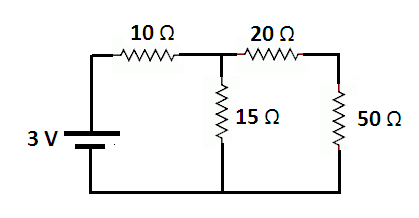Solution:

• To solve the above circuit, mark points A, B, C, D, E, F.
• Apply Kirchhoff’s Current Law at the junction B, mark the assumed direction of flow of current in various branches i.e. Incoming currents = Outgoing currents.
• In this problem, there are two unknown quantities (I1 and I2). Therefore, we have to choose two closed circuits to solve the problem.

The current is starting from the positive plate of the 3 V cell is I1. This current after flowing in 10 Ω resistance, is divided into two parts at point B.

One part I2 goes through 20 Ω resistance and 50 Ω resistances and the remaining part I1 – I2 goes through 15 V Ω resistance.

Both the currents meet at point E and again current I1 reaches the negative plate of the cell.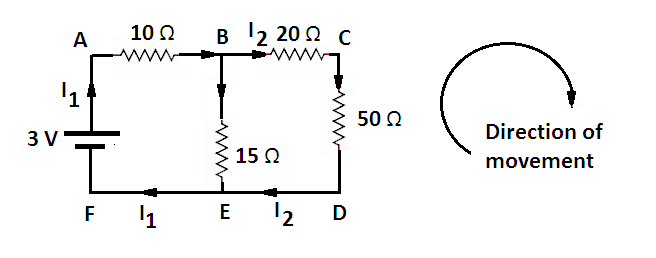Consider the closed path ABEFA.

If we trace the closed path in the direction ABEFA, various voltage drops and EMF’s will have the following signs:

• Voltage drop in 10 Ω = 10 I1 negative (fall in potential).
• Voltage drop in 15 Ω = 15(I1 – I2) negative (fall in potential).
• EMF = 3 positive (rise in potential).

Applying Kirchhoff’s Voltage Law to mesh ABEFA, we get,

– 10 I1 – 15(I1 – I2) + 3 = 0

or – 10 I1 – 15 I1 + 15 I2 +3 = 0
or – 25 I1 + 15 I2 + 3 = 0

or 25 I1 – 15 I2 – 3 = 0 ………. (i)

Now to get the second equation, consider the closed path BCDEB.

If we trace the closed path in the direction BCDEB, various voltage drops and EMF’s will have the following signs:

• Voltage drop in 20 Ω = 20 I2 negative (fall in potential).
• Voltage drop in 50 Ω = 50 I2 negative (fall in potential).
• Voltage drop in 15 Ω = 15(I1 – I2) positive (rise in potential).
• EMF = 0 (no EMF source in this mesh).

Applying Kirchhoff’s Voltage Law to mesh BCDEB, we get,

– 20 I2 – 50 I2 + 15(I1 – I2) + 0 = 0

or – 20 I2 – 50 I2 + 15 I1 – 15 I2) = 0
or – 85 I2 + 15 I1 = 0
or – 17 I2 + 3 I1 = 0

or 3 I1 – 17 I2 = 0 ………..( ii)

By solving equations (i) and (ii), we get,

I1 = 0.134 A and
I2 = 0.024 A

Example: A circuit is shown in Figure. Calculate the current in each branch of the circuit.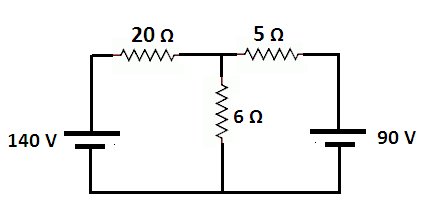Solution:

• To solve the above circuit, mark points A, B, C, D, E, F.
• Apply Kirchhoff’s Current Law at the junction B, mark the assumed direction of flow of current in various branches i.e. Incoming currents = Outgoing currents.
• In this problem, there are two unknown quantities (I1 and I2). Therefore, we have to choose two closed circuits to solve the problem.

Consider the closed path ABEFA.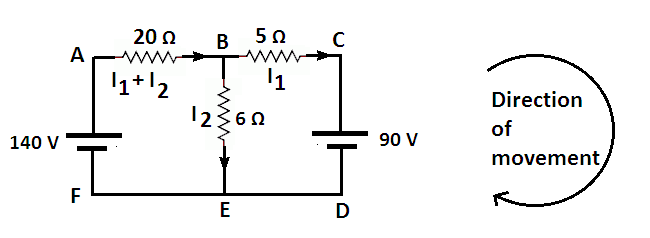If we trace the closed path in the direction ABEFA, various voltage drops and EMF’s will have the following signs:

• Voltage drop in 20 Ω = 20(I1 + I2) negative (fall in potential).
• Voltage drop in 6 Ω = 6 I2 negative (fall in potential).
• EMF = 140 positive (rise in potential).

Applying Kirchhoff’s Voltage Law to mesh ABEFA, we get,

– 20(I1 + I2) – 6 I2 + 140 = 0
or 20 I1 + 26 I2 = 140
or 10 I1 + 13 I2 = 70 …….. (i)

Now, to get the second equation, consider the closed path BCDEB.

If we trace the closed path in the direction BCDEB, various voltage drops and EMF’s will have the following signs:

• Voltage drop in 5 Ω = 5 I1 negative (fall in potential).
• Voltage drop in 6 Ω = 6 I2 positive (rise in potential).
• EMF = 90 negative (fall in potential).

Applying Kirchhoff’s Voltage Law to mesh BCDEB, we get,

– 5 I1 – 90 + 6 I2 =0
or – 5 I1 + 6 I2 = 90   ………. (ii)

By solving equations (i) and (ii), we get,

I2 = 10 A
I1 = – 6 A

Therefore, current in branch BE is 10 A, current in branch BC is 6 A (but opposite to the assumed direction, because I1 has a negative sign in the solution) and current in branch AB is 16 A.